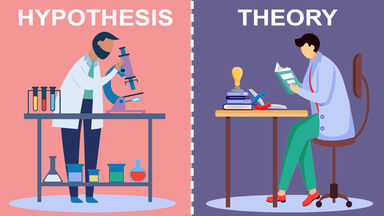# Primary Difference Between Hypothesis and Theory

The primary difference between a hypothesis and a theory is the point at which they are formed in the scientific process. A hypothesis is made before an experiment while a theory is formed after collecting a lot of scientific evidence. Explore how these terms differ from each other and similar science terms.difference between hypothesis and theory

## Definition of Hypothesis

In the scientific process, a hypothesis is “a statement that makes generalizations about a set of facts, usually forming a basis for possible experiments” or, “an unproven theory or statement.”

Students often learn that a hypothesis is an educated guess. It is basically an assumption about what might happen based on known information that is used for the sake of argument when testing something.

## Definition of Theory

A theory is “an idea to explain something or a set of guiding principles” or, ”a set of statements or principles devised to explain a group of facts or phenomena, especially one that has been repeatedly tested or is widely accepted.”

A theory is an explanation for why or how something happens that is backed by a lot of data collected over time and from different circumstances.

## Hypothesis vs. Theory

Based on definitions alone, you can see that the main difference between a hypothesis and a theory is that a hypothesis is one statement while a theory is a set of principles or statements.

### Main Differences Between Hypothesis and Theory

More specific differences between these two terms include the following:

• A hypothesis is typically formed before an experiment, while a theory is typically formed after several experiments or studies have been performed.
• A hypothesis is one statement; a theory is a collection of things.
• A theory is more likely to be true than a hypothesis.
• A hypothesis is usually tentative, while a theory is typically more permanent.
• A theory is always backed by evidence, but a hypothesis doesn’t have to be.
• A hypothesis is specific to a single test or observation, while a theory is a general principle that can work in multiple instances.

### Examples of Theory vs. Hypothesis

If you look at examples of famous theories in science, you can see the differences between the complete theories and what could have been the hypotheses of the men who created them.

 Theory Hypothesis Oxygen Theory of Combustion Phlogiston does not play a role in combustion. Darwin’s Theory of Evolution Organisms better adapted to their environments will survive and produce more offspring. Heliocentric Theory The Earth and other planets revolve around the sun.

### Similarities Between Hypothesis and Theory

Both theories and hypotheses are usually based on some date and can be tested. This means either could be substantiated or falsified.

### Non-Scientific Use of Theory and Hypothesis

In common speech, people use theory and hypothesis as synonyms referring to something they speculate. You might say, “I have a theory about where my dog is hiding all my shoes.” This wouldn’t be proper use of the term, but it is acceptable in normal conversation.

## Hypothesis vs. Theory vs. Law

A law is “a sequence of events that has been observed to occur with unvarying uniformity under the same conditions.” Any scientific principle that has been shown true over and over again, and is therefore accepted without question by most, is a law. A hypothesis is not as firm as a law, although a theory can be.

## Get Your Theory Straight

In the scientific method, a hypothesis comes before a theory because you need evidence to support a theory and that evidence typically comes from tests that start with hypotheses. Explore more commonly confused science terms like hypothesis vs. prediction or data vs. information.